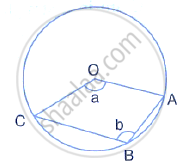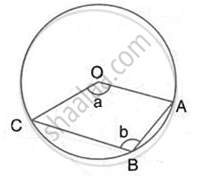Share

The Figure Given Below, Shows a Circle with Centre O. Given: ∠Aoc = a and ∠Abc = B. (I) Find the Relationship Between a and B (Ii) Find the Measure of Angle Oab, is Oabc is a Parallelogram - ICSE Class 10 - Mathematics

ConceptArc and Chord Properties - the Angle that an Arc of a Circle Subtends at the Center is Double that Which It Subtends at Any Point on the Remaining Part of the Circle

Question

The figure given below, shows a Circle with centre O.
Given: ∠AOC = a and ∠ABC = b.
(i) Find the relationship between a and b
(ii) Find the measure of angle OAB, is OABC is a parallelogramSolution(i) ∠ABC = 1/2Reflex (COA)

(Angle at the centre is double the angle at the circumference subtended by the same chord)

⇒ b = 1/2 (360 -a)

⇒ a + 2b = 180°

(ii) Since OABC is a parallelogram, so opposite angles are equal
∴ a = b
Using relationship in (i)
3a = 180°
a = 60°

Also, OC || BA
∴ ∠COA + ∠OAB = 180°
⇒ 60°  + ∠OAB = 180°
⇒ ∠OAB = 120°

Is there an error in this question or solution?

Video TutorialsVIEW ALL 

Solution The Figure Given Below, Shows a Circle with Centre O. Given: ∠Aoc = a and ∠Abc = B. (I) Find the Relationship Between a and B (Ii) Find the Measure of Angle Oab, is Oabc is a Parallelogram Concept: Arc and Chord Properties - the Angle that an Arc of a Circle Subtends at the Center is Double that Which It Subtends at Any Point on the Remaining Part of the Circle.
S